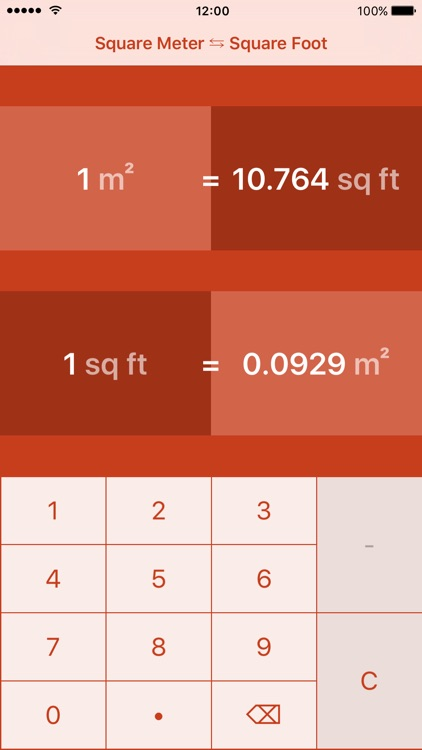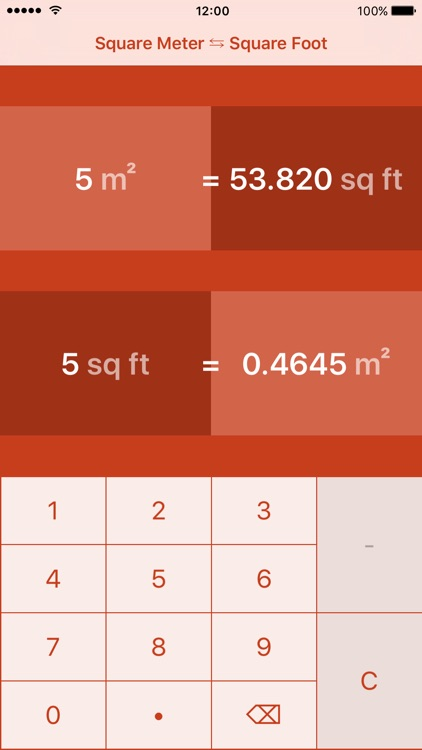## Quickest and easiest way to convert between square meter and square foot# Square Meters To Square Feet | m² to ft²

by Meta Technologies GmbHQuickest and easiest way to convert between square meter and square foot.### App Details

Version
2.0.0
Rating
NA
Size
10Mb
Genre
Utilities Education
Last updated
June 30, 2016
Release date
December 14, 2015

### App Store Description

Quickest and easiest way to convert between square meter and square foot.

Features:
- Easy to use
- Convert in both directions
- No InAppPurchases

Alternative spelling:
- convert square meter to square foot
- convert square meters to square foot
- convert square meter to square feet
- convert square meters to square feet
- convert square meter to ft²
- convert square meters to ft²
- convert m² to square foot
- convert m² to square feet
- convert m² to ft²
- convert square meter in square foot
- convert square meters in square foot
- convert square meter in square feet
- convert square meters in square feet
- convert square meter in ft²
- convert square meters in ft²
- convert m² in square foot
- convert m² in square feet
- convert square meter into square foot
- convert square meters into square foot
- convert square meter into square feet
- convert square meters into square feet
- convert square meter into ft²
- convert square meters into ft²
- convert m² into square foot
- convert m² into square feet

Disclaimer:
AppAdvice does not own this application and only provides images and links contained in the iTunes Search API, to help our users find the best apps to download. If you are the developer of this app and would like your information removed, please send a request to [email protected] and your information will be removed.